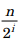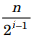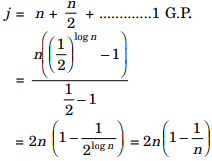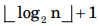# Data Structures and Algorithms - Performance Analysis of Algorithms and Recurrences

>>>>>>>>Performance Analysis of Algorithms and Recurrences

• A

O(1)• B

O(n)• C

O(n!)• D

O(nn)• Option : B
• Explanation :
Here foo(1) is recursive function. Spare complexity is O (n) as there can be at most O (n) active function at a time

• A

O(1)• B

O(n)• C

O(n2)• D

O(n!)• Option : B
• Explanation :
Space complexity is O (n). The space we need array of size O (n). The space required for recursive call would be O (1) as the value would be taken from array again & again

• A

val(j) = Θ(logn)• B

val(j) = Θ(√n)• C

val(j) = Θ(n)• D

val(j) = Θ(nlogn)• Option : C
• Explanation :
If n is a power of 2, thenterm
If n is not a power of 2, then there will be minor differences of 1 atwhereveris odd.
Hence val(j) computed on the basis of n = 2n will give a fair answer= 2(n – 1) = θ(n)

• A

T(n) = Θ(log log n)• B

T(n) = Θ(log n)• C

T(n) = Θ(√n)• D

T(n) = Θ(n)• Option : B
• Explanation :
T(n) = 2T(√n) + 1
Put n = 2k
T(2k) = 2T(nk/2) + 1
replace T(2k) by S(k)
S(k) = 2 S(k/2) + 1
Apply masters
Klog22 ⇒ k > 1
So Θ(k)
Now we know n = 2k, k = log2(n)
then Θ(logn)

• Option : D
• Explanation :
Since, j increases in power of 2's.
if statement j = j * 2 executes k times, then
2k < n
⇒ k < log2 n
Since k will be integer,
total number of comparison(when loop exits)